## Tuesday, January 01, 20192014
(May)
MATHEMATICS
(Major)
Course: 401
[A: Computer Programming (C Programming)]
Full Marks: 50
Pass Marks: 20
Time: 2 ½ hours
The figures in the margin indicate full marks for the questions

1. Choose the correct answer: 1x5=5
1. The statement char Ch = ‘Z’ would store in ch
1. The character Z.
2. ASCII value of Z.
3. Z along with the single inverted commas.
4. None of the above.
1. Which of the following is not an arithmetic operator in C?
1. +
2. /
3. **
1. To receive the string “We have got the guts, you get the glory II” in an array char str , which of the following functions would you use?
1. Scanf (“% s”, str);
2. Gets (str);
3. Getch (str);
4. Fgetchar (str);
1. The ‘break’ statement is used to exist from
1. An if statement.
2. A for loop.
3. A program.
4. The main ( ) function.
1. Which of the following is not a valid return statement in C?
1. Return (a);
2. Return (23);
3. Return;
4. Return (a, b);
2. Answer any eight of the following questions: 2x8=16
1. Write a short note on flowchart.
2. Mention the storage classes in C.
3. Mention the relational operators in C.
4. Evaluate the following expression assuming that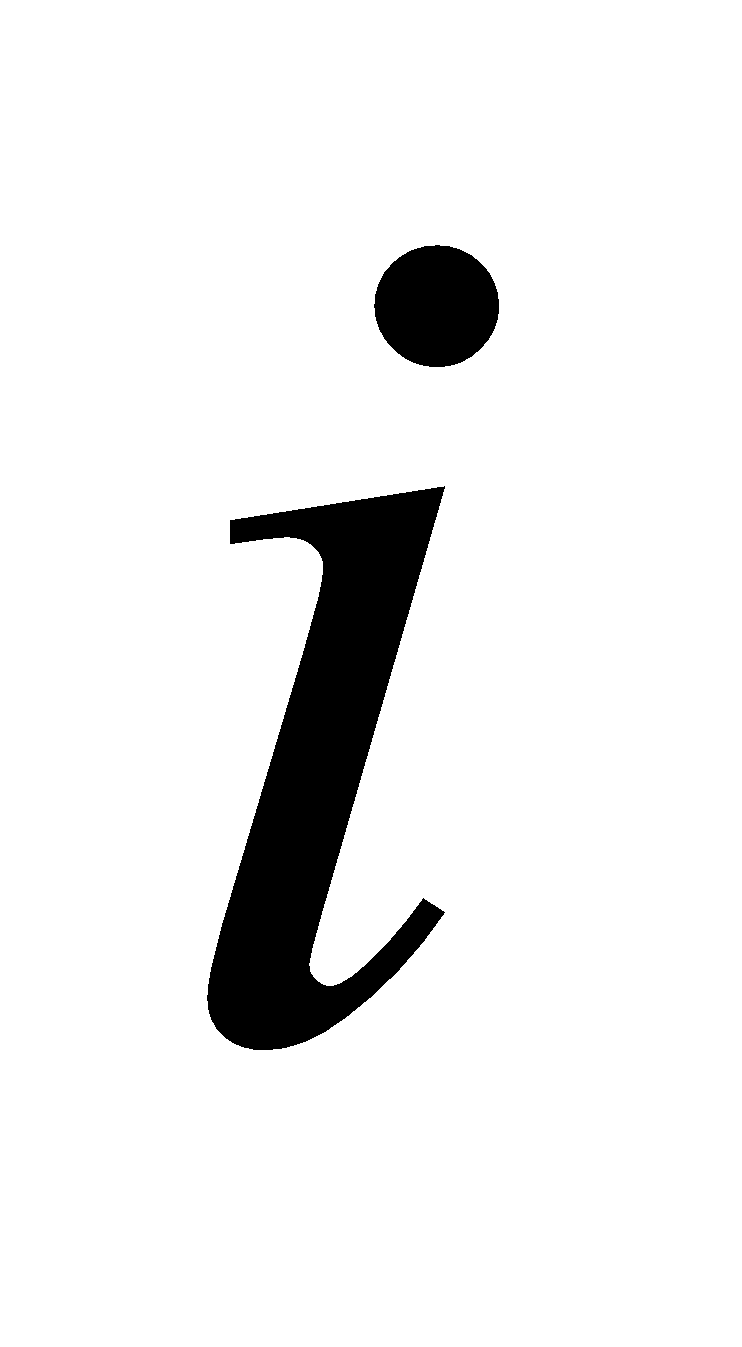is an integer variable: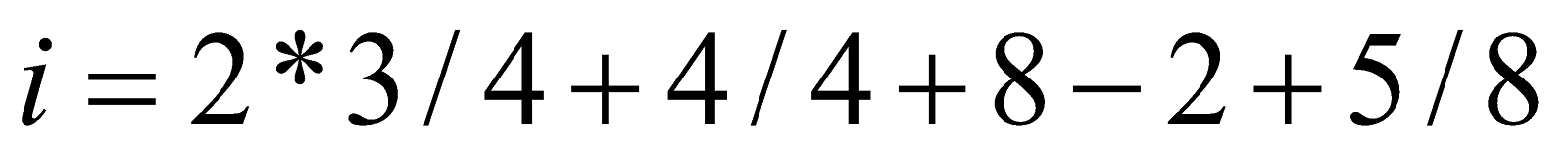1. Convert the equation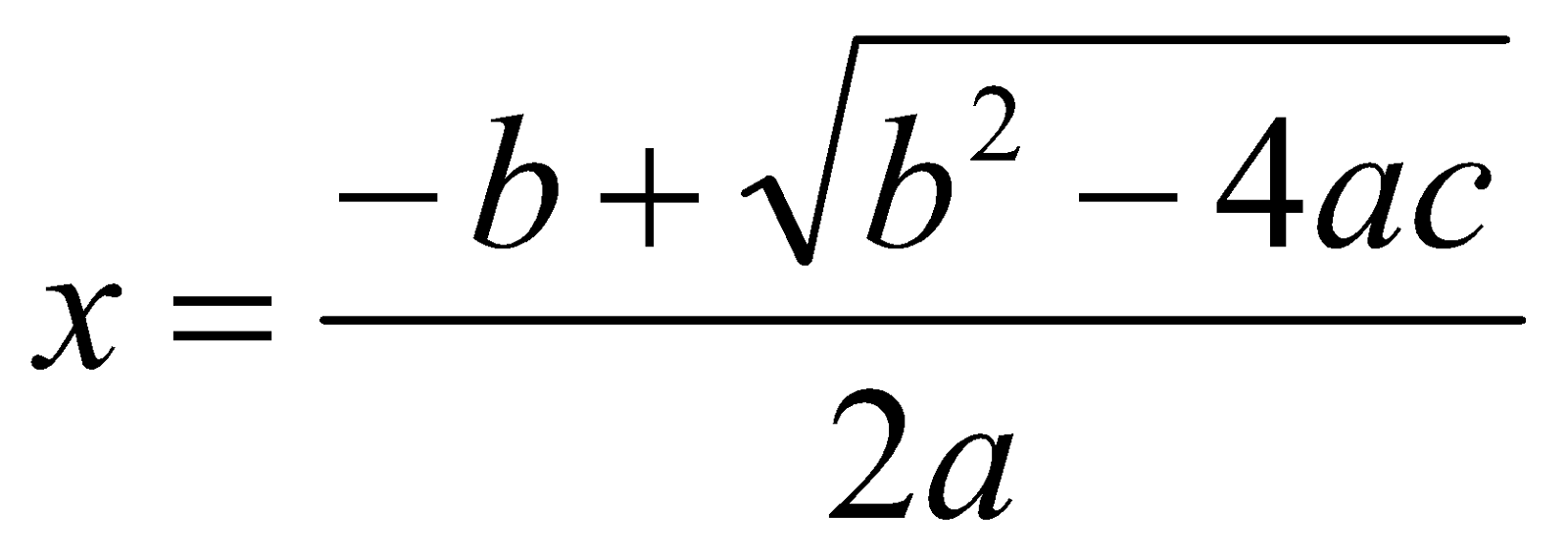into corresponding C statement.

1. Distinguish between the following pairs:
1. % s and % c specifications for reading.
2. % f and % d specifications for printing.
1. Write a C program to evaluate the area of a triangle.
2. Any integer is input through the keyboard. Write a C program to find out whether it is an odd number or even number.
3. Write a C program to print the numbers from 1 to 10.
4. Write a C program to read and print a 2 x 2 matrix.
3. What is algorithm? Write the algorithm to evaluate the area and perimeter of a rectangle. 1+2=3
4. Temperature of a city in Fahrenheit degrees is input through the keyboard. Write a C program to convert this temperature into Centigrade degrees.
Or
Ramesh’s basic salary is input through the keyboard. His dearness allowance is 40% of basic salary and house rent allowance is 20% of basic salary. Write a C program to calculate his gross salary.
5. The marks obtained by a student in 5 different subjects are input through the keyboard. The student gets a division as per the following rules:
Percentage above or equal to 60 – First Division
Percentage from 50 to 59 – Second Division
Percentage from 40 to 49 – Third Division
Percentage less than 40 – Failed.
Write a C program to calculate the division obtained by the student. 4
Or
Write a C program to read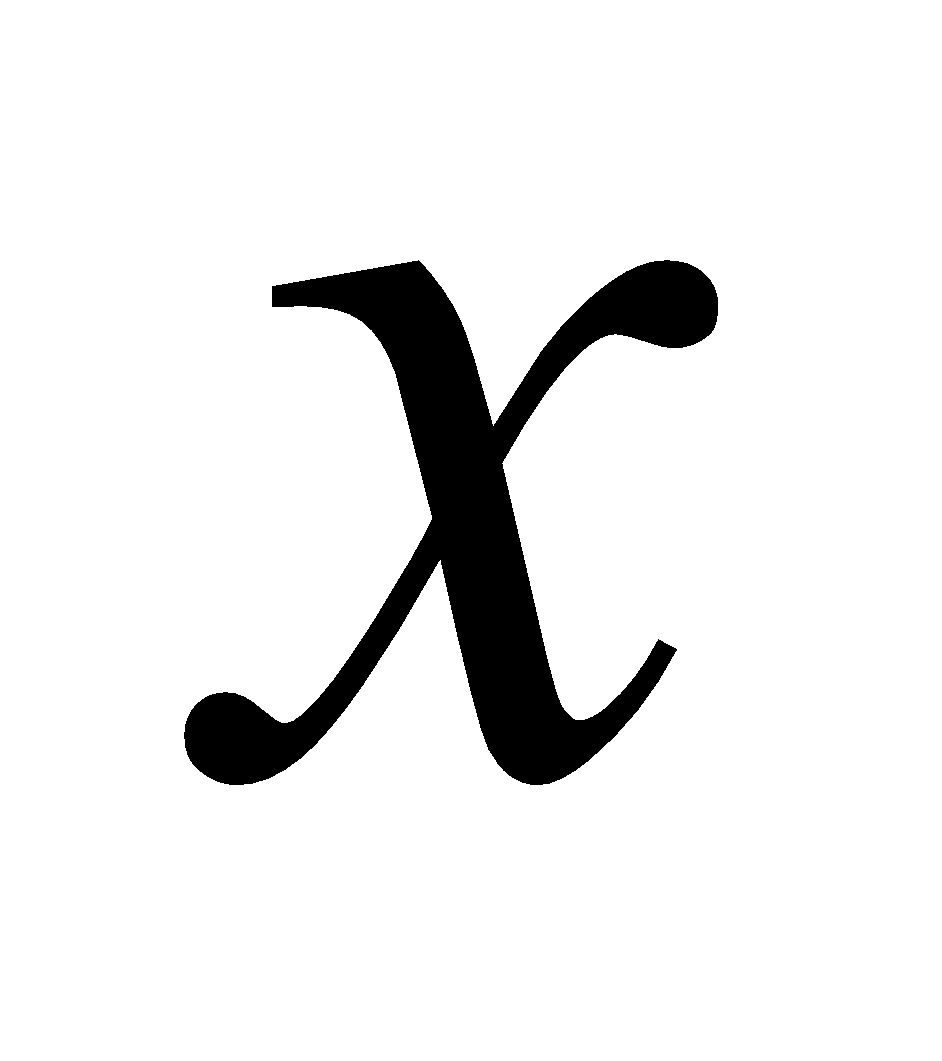and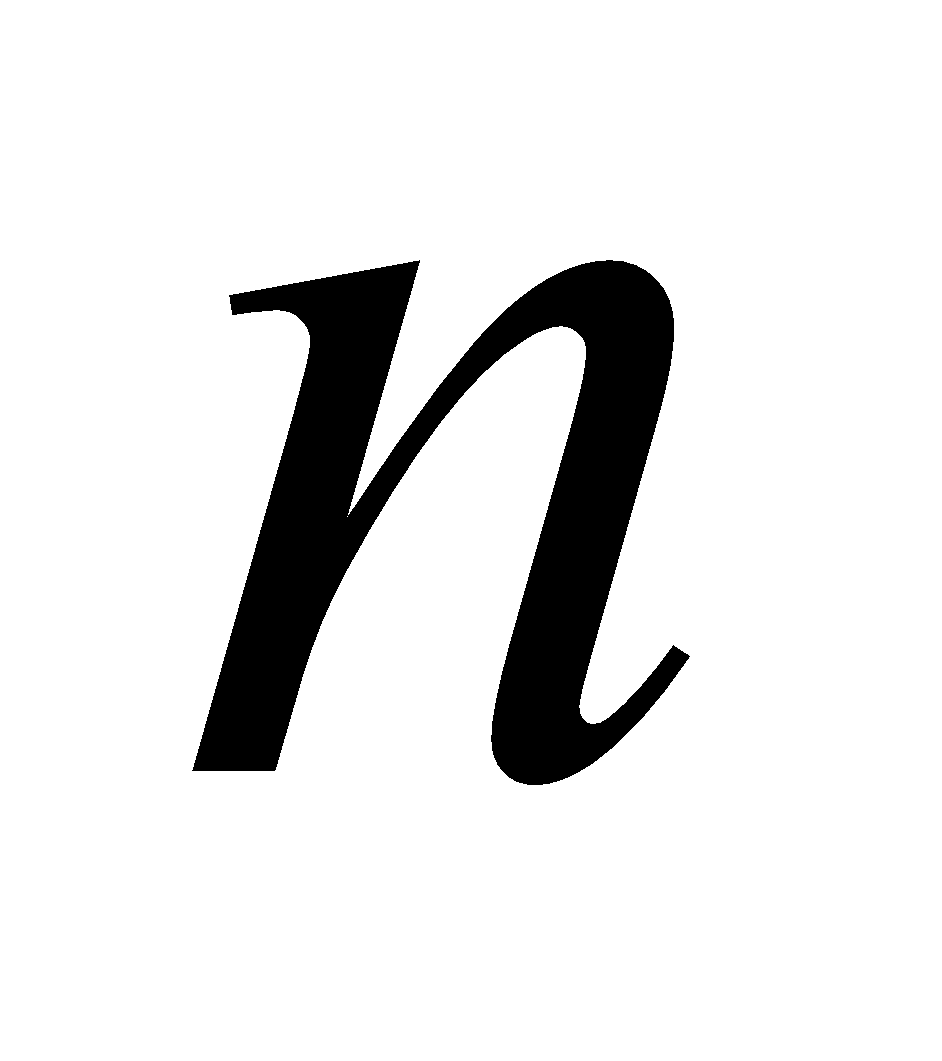, and evaluate the sum of the series6. Define an array. Write a C program to evaluate total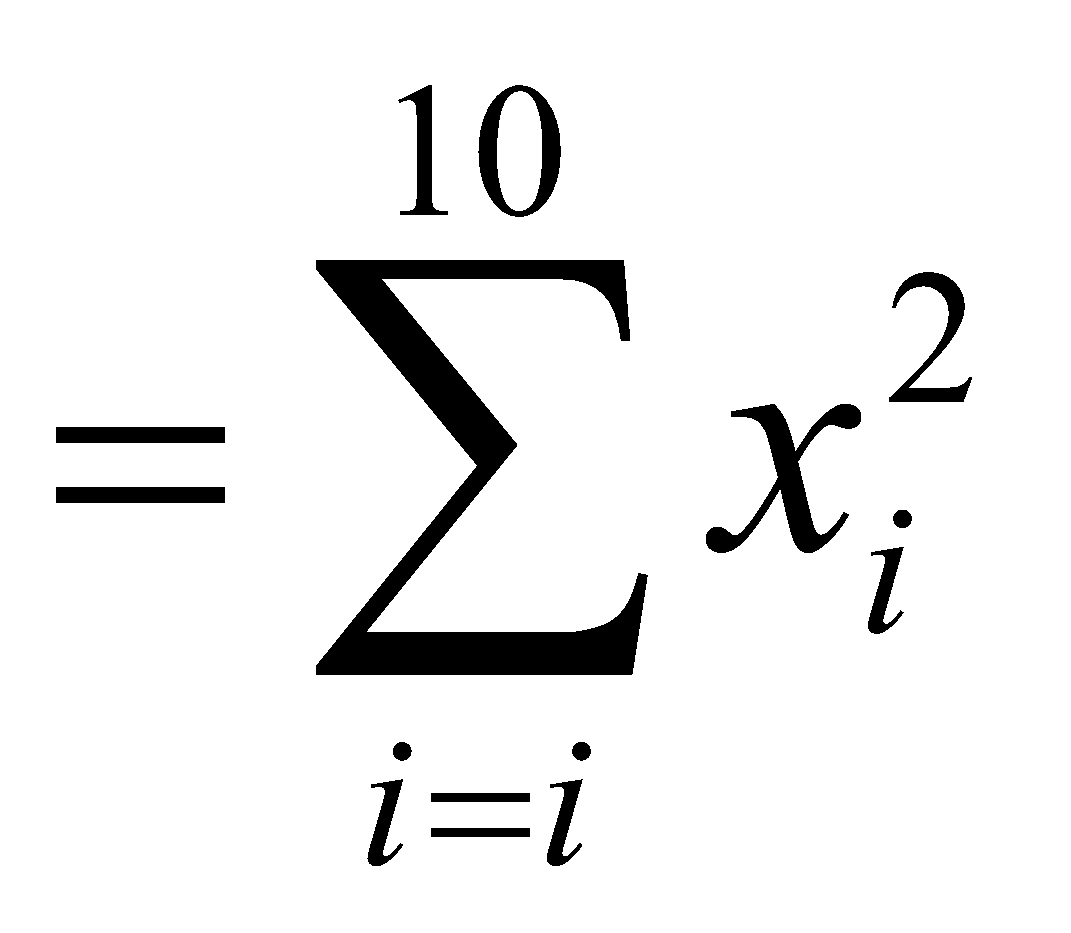1+3=4
Or
Write a C program to add two matrices of order 3 x 3. 4
7. Write a C program to choose minimum of a one-dimensional array of3
Or
Write a C program to calculate the sum of positive even numbers less than or equal to 100.
8. What do you mean by user defined functions? Write a C program to find the sum of two integers using function prototype. 1+3=4
Or
Write a C program to calculate the sum of the digits of a five-digit integer number. 4
9. Write a C program to arrange an array of 20 numbers in ascending order using function prototype. 4
Or
Write a C program to evaluate a real root of the equation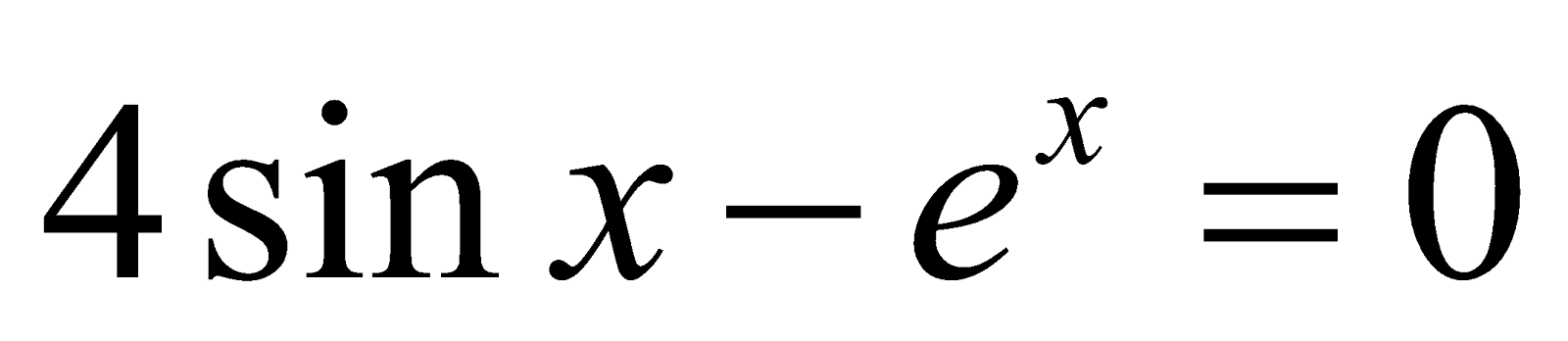using Newton-Raphson method.
10. Write a C program to find the sum of the sine series up toterms. It is given that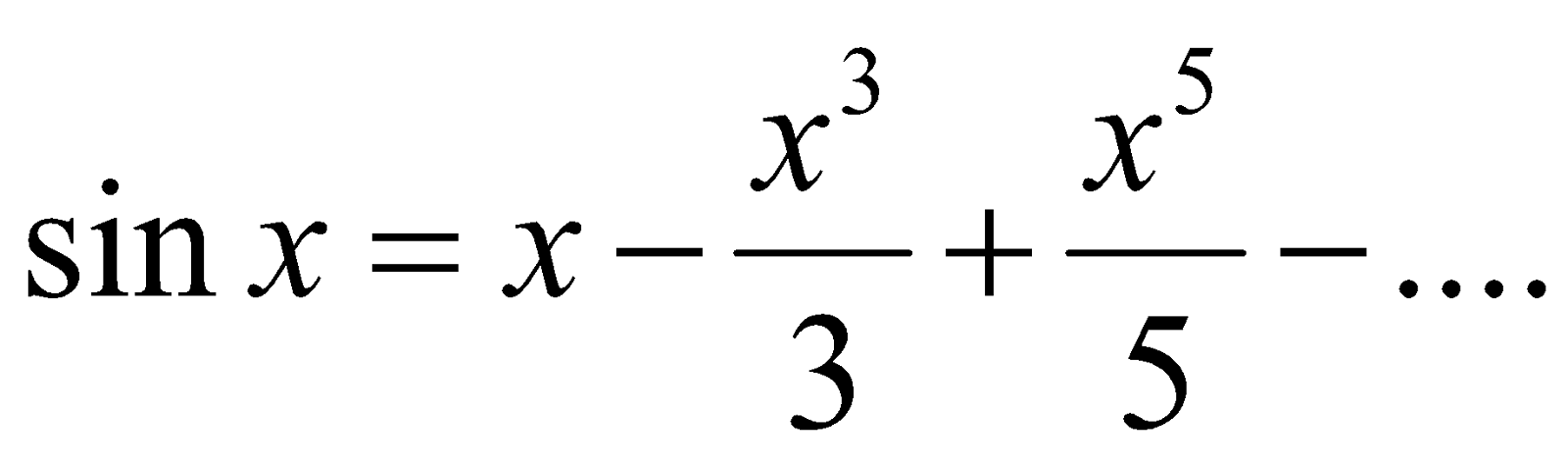Write a C program to multiply two matrices of orders 6 x 6.

***